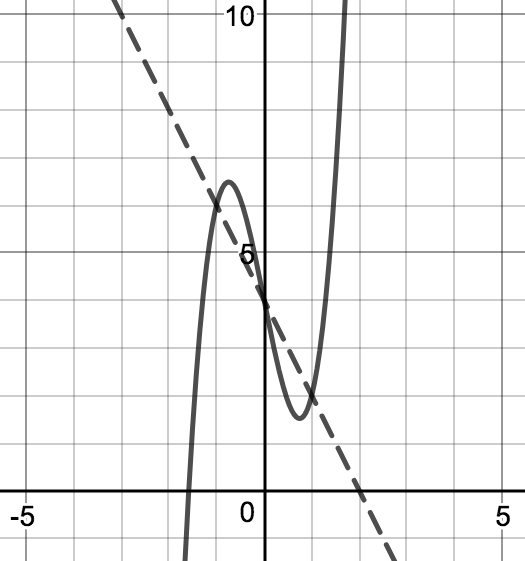# Polynomials

## Objective

Identify the solution(s) to systems of polynomial functions.

## Common Core Standards

### Core Standards

?

• A.REI.D.11 — Explain why the x-coordinates of the points where the graphs of the equations y = f(x) and y = g(x) intersect are the solutions of the equation f(x) = g(x); find the solutions approximately, e.g., using technology to graph the functions, make tables of values, or find successive approximations. Include cases where f(x) and/or g(x) are linear, polynomial, rational, absolute value, exponential, and logarithmic functions. Modeling is best interpreted not as a collection of isolated topics but in relation to other standards. Making mathematical models is a Standard for Mathematical Practice, and specific modeling standards appear throughout the high school standards indicated by a star symbol (★). The star symbol sometimes appears on the heading for a group of standards; in that case, it should be understood to apply to all standards in that group.

?

• A.REI.D.10

## Criteria for Success

?

1. Explain that the solution to a system of polynomial functions represents the intersection of the two functions.
2. Describe that when two functions are set equal to one another you can solve them as a system of equations, and this solution can be seen graphically and proven algebraically.
3. Identify the procedures necessary to solve a system of equations, including factoring or use of quadratic formula.
4. Describe that, in basic terms, you need the same number of equations as variables.

## Anchor Problems

?

### Problem 1

What are the intersections of the functions ${f(x)=x^2}$ and ${g(x)=x^4}$?

#### References

Illustrative Mathematics Graphs of Power Functions

Graphs of Power Functions, accessed on Sept. 25, 2017, 2:44 p.m., is licensed by Illustrative Mathematics under either the CC BY 4.0 or CC BY-NC-SA 4.0. For further information, contact Illustrative Mathematics.

### Problem 2

Below is a graph of the function ${h(x)=3x^3-5x+4}$ and a graph of the function ${j(x)=-2x+4}$. For what values does ${h(x)=j(x)}$? Explain your reasoning algebraically and graphically.## Problem Set

?

The following resources include problems and activities aligned to the objective of the lesson that can be used to create your own problem set.

Using your graphing calculator, find the values of $x$ for which $h(x)=m(x)$.
$h(x)=2x^3+2x^2-x+3$
$m(x)=(x-4)^2-3$